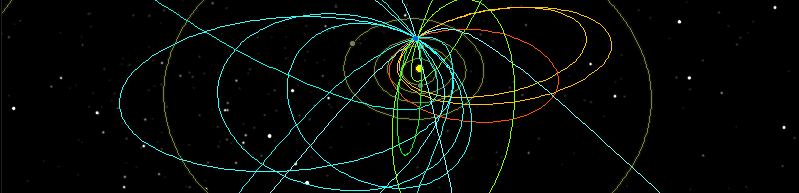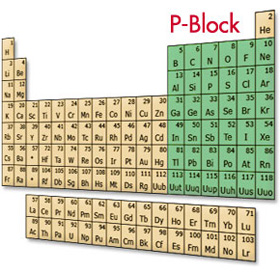## LIFE H#RMONIZER

### THE NEXT WAVE IN PERSONAL CAREOrbitals & Electrons

An orbital is a region of probability in which the electron can be found. An orbital, like a loveseat, can hold up to two occupants, in this case electrons. The loveseats can be different "styles" based on the energy of the electrons that will occupy them and the energy level they are found in.

The lowest-energy "style" orbital is the s orbital. It is sphere-shaped. All of the elements in the first 2 columns on the left side of the Periodic Table are filling the s orbital of their highest energy level with their final electron. This makes the first 2 columns of the table the s block of elements.

The S Orbital

The sphere shaped s orbital is the first place in an almost empty space where an electron can orbit an atom's nucleus.

Hydrogen has 1 proton and thus 1 electron, going into the 1st energy level, in the 1s orbital. Helium has 2 protons, thus 2 electrons, being placed 1 at a time into the 1s orbital.

This completes the first ring and balances this energy level. Therefore helium is not reactive, and as such the first of a group of elements referred to as the inert or noble gases.The P Orbital

Once the s orbital has been filled for that energy level, the remaining electrons start filling the next orbital place, called the p orbital. The p orbitals are shaped like propeller blades, with the 1st set lined up along the atom's X axis (horizontal).

A 2nd p orbital is lined up along the atom's Y axis (vertical). The 3rd and final p orbital is lined up along the atom's Z axis (front to back).

This makes a total of 3 p orbitals to be filled in any given energy level from the 2nd up. Each of these 3 p orbitals needs to be filled with electrons in the 2nd and higher energy levels. But there is another rule to follow in placing the electrons in the p orbitals.

This is Hund's Rule. It states that each p orbital must receive 1 electron before any p orbital can receive a 2nd electron.

Filling the Orbitals

The boron atom has 5 protons and thus 5 electrons. The first 2 electrons are placed into the 1s orbital. The 3rd, and 4th electrons are placed in the 2nd energy level. Here, the 1st orbital to be filled is the 2s orbital. The last electron goes into a p orbital. Boron is the first element in the periodic table that has an electron in the "p block."

It is made up of the elements that are filling p orbitals with their final electrons. In the table, there is a rectangular segment located to the right of the drop-down central portion. There are 6 columns of elements in this rectangle. These are the elements with filled p orbitals, the p block. (Helium is an exception, as the first energy level does not have any p orbitals.)The D Orbital

There are 2 other "styles" of orbitals that are too complex to go into detail about here. They are the d orbitals, of which there are 5, and the f orbitals, of which there are 7.

The Periodic Table provides a section for each of these groups of orbitals. The 10 electrons of the 5 d orbitals are filled by elements found in the table's dropped central part.

This section is called the d block elements, or the transition metals. The 2 rows found separated at the bottom of the table are the elements of the f block. These elements fill the highest energy levels of the f orbitals when they place their last and highest-energy electrons at the ground state.

Conclusion

The presence of the Life Harmonizer field catalyzes the body to re-condition the quantum particles inside each of the molecules in all of our trillions of cells.

This way, the original quantum field is gradually recreated throughout the 3.5 billion rungs of the DNA double helix ladder in each of these trillions of cells, and thus further
harmonize our life